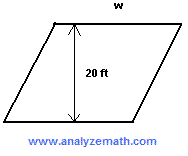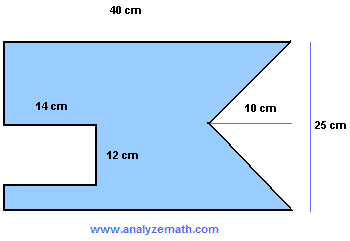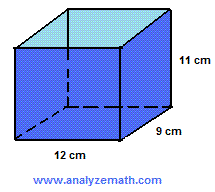Geometry problems and questions with answers for grade 8 are presented. These problems and questions deal with calculating angles, perimeters, areas and volumes. Questions on similarity and reflection are also included. Also solutions and explanations are included.

1. Find the total surface area and the volume of a closed cylindrical container with radius 5 cm and a height of 34 cm.

2. Find the total surface area and the volume of a closed conical container with radius 5 cm and a height of 15 cm.(round your answer to the nearest unit.)

3. A cube has a total surface area of the six faces equal to 150 square feet. What is the volume of the cube?

4. Which two angles are complementary?

1. 21 degrees and 78 degrees
2. 58 degrees and 22 degrees
3. 67 degrees and 23 degrees
4. 140 degrees and 40 degrees

5. Which two angles are not supplementary?

1. 30 degrees and 150 degrees
2. 5 degrees and 175 degrees
3. 89 degrees and 91 degrees
4. 23 degrees and 177 degrees

6. Find the height h of the trapzoid so that its area is equal to 400 square cm..

7. Find the width w of the parallelogram so that its area is equal to 600 square feet..

8. Find area of the shaded shape..

9. Find the total surface area of the box open at the top..

10. If the quadrilateral ABCD is a parallelogram, what are the coordinates of point D?.

11. Which of these are right triangles?.

12. Find x if triangle ABC is a right triangle with angle B = 90�..

13. Find all the unknown sides x, y, z and w if all three triangles are similar..

14. A quadrilateral with vertices (-2,6) , (6,8) , (9,2) and (4,-1) is reflected on the x axis. What are the coordinates of the vertices of the quadrilateral after reflection?

15. The side of cube A is 3 times the side of cube B. The volume of cube A is 3,375 cubic feet. Find the volume of cube B.

16. The length of rectangle A is 24 cm and the length of rectangle B is 96 cm. The two rectangles are similar. Find the ratio of the area of A to the area of B.

## Answers to the Above Questions

1. area = 390 pi square cm , volume = 850 pi cubic cm
2. area = 327 square cm , volume = 393 cubic cm
3. volume = 125 cubic feet
4. C) 67 degrees and 23 degrees are complementary
5. D) 23 degrees and 177 degrees are not supplementary
6. h = 20 cm
7. w = 30 feet
8. area = 707 square cm
9. surface area = 570 square cm
10. (1 , 2)
11. triangles A) and C) are right triangles
12. x = 102 o
13. x = 9 , y = 51 , z = 144 , w = 36
14. vertices after reflection (-2,-6) , (6,-8) , (9,-2) and (4,1)
15. volume of cube B = 125 cubic feet
16. ratio of area of A to area of B = 1:16